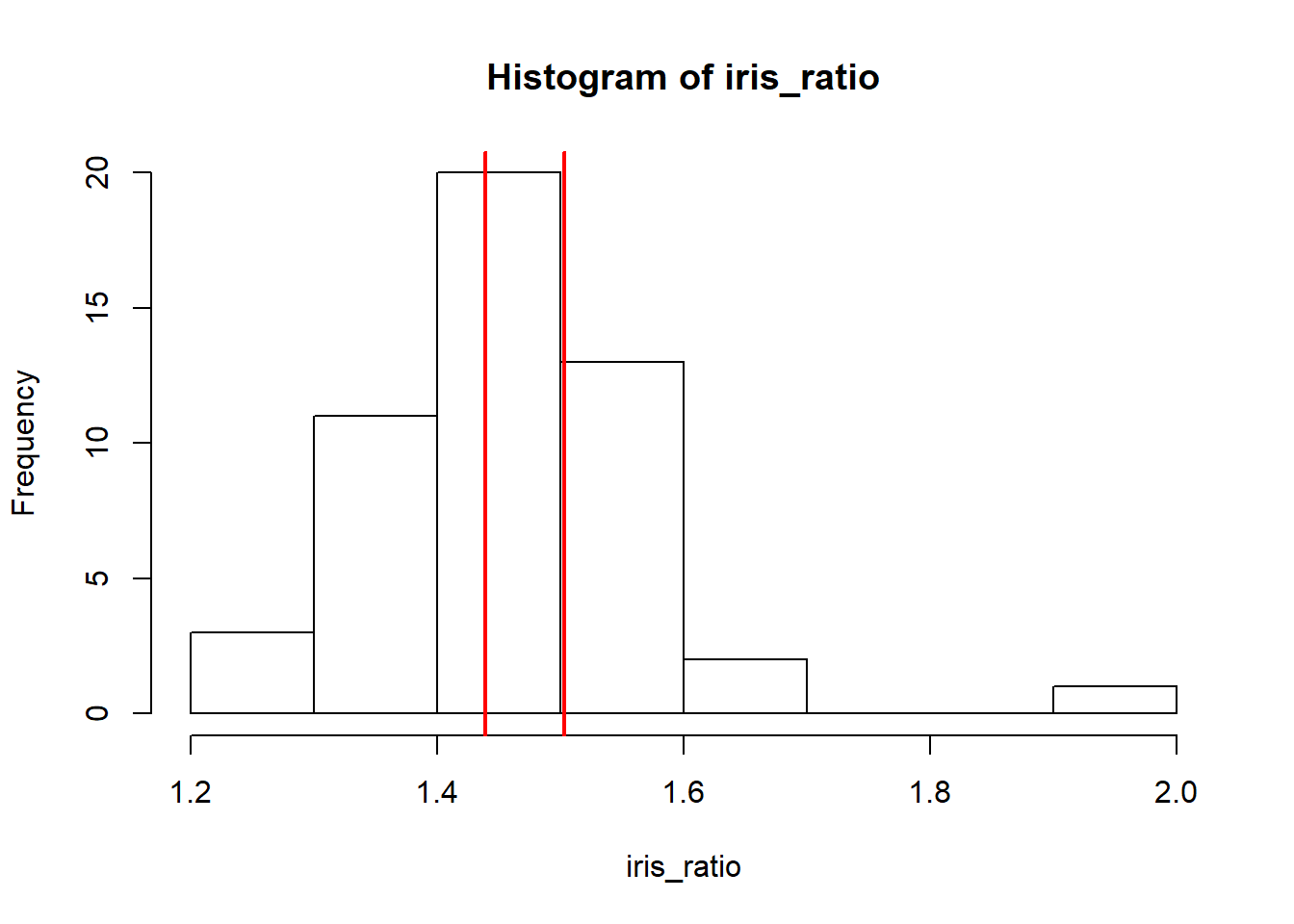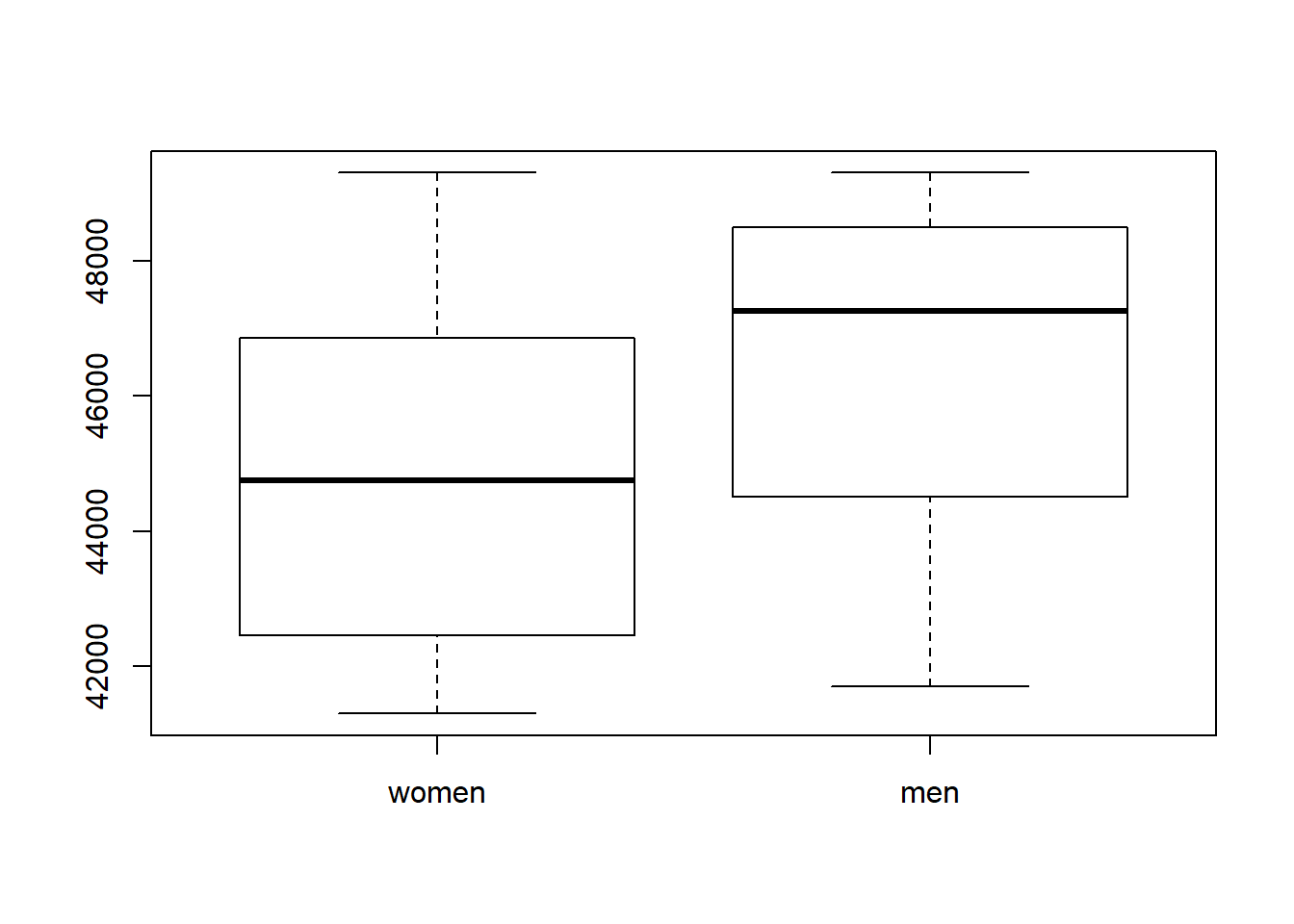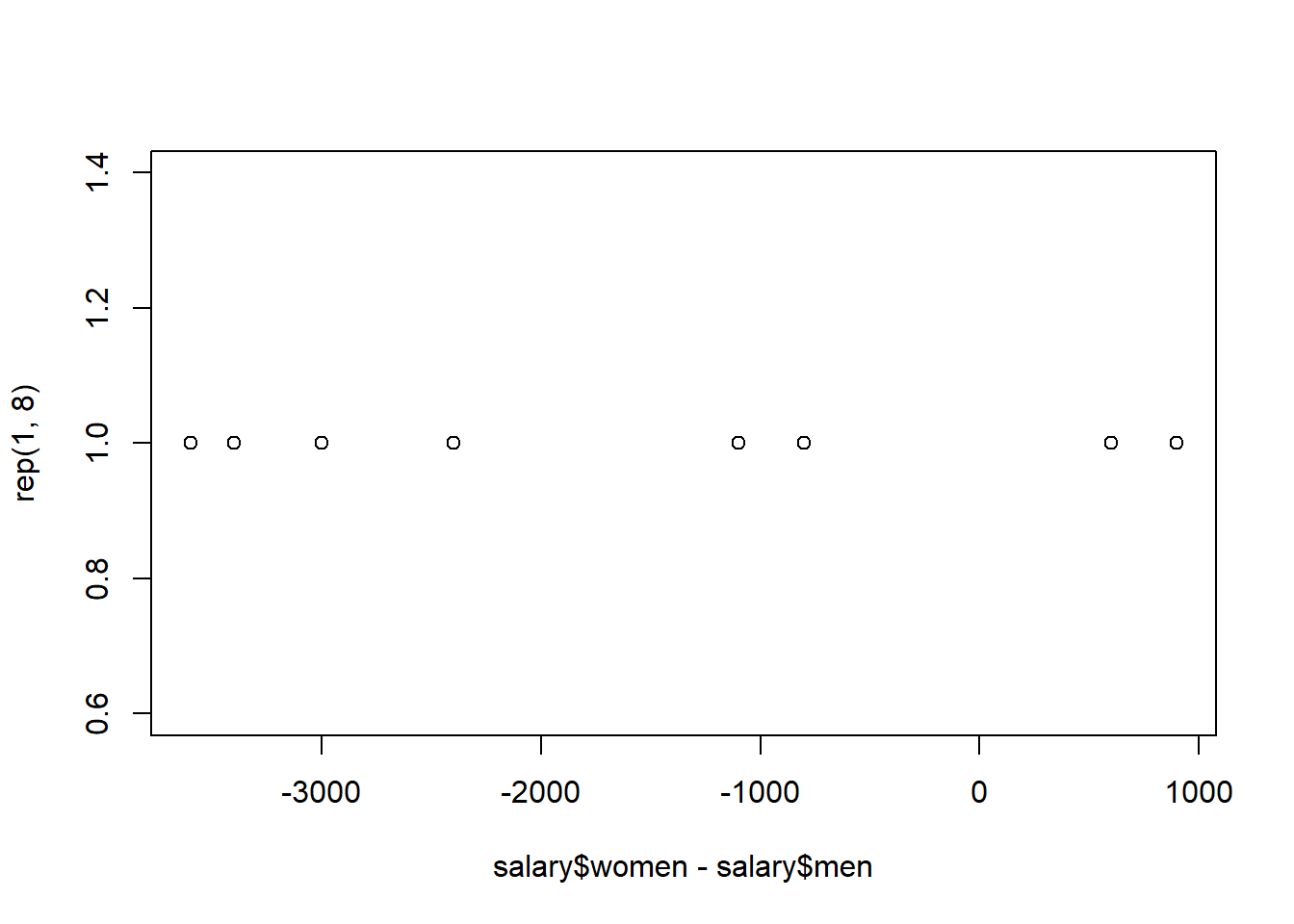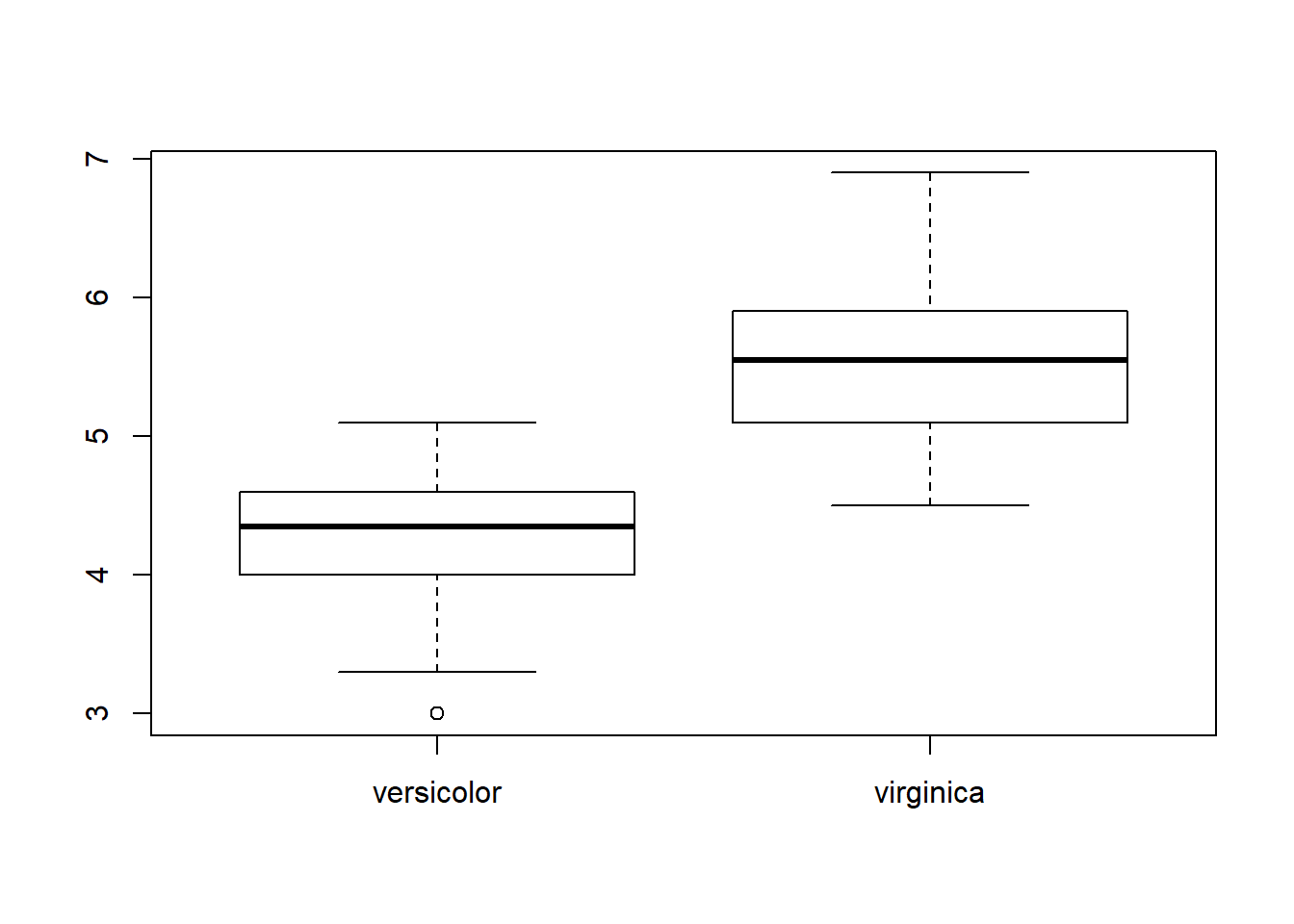### Homework exercise

To be solved at home before the exercise session.

1. Consider the confidence interval for the expected value of the normal distribution on page 2.9 of the lecture notes. Describe what will (most likely) happen to the width of the confidence interval (does it get smaller, larger or stay the same?) if we,
1. Increase the sample size $$n$$.
2. Decrease the confidence level $$100(1 - \alpha)$$.
3. Increase the variance $$\sigma^2$$.
4. Decrease the expected value $$\mu$$.
# a.
# The width of the interval is proportional to $1/\sqrt{n}$, and thus increasing $n$ will make the interval shorter.
# Heuristic explanation: larger sample = more information = larger precision = shorter interval.

# b.
# Decreasing $100(1 -\alpha)$ (increasing alpha) will decrease the corresponding quantile of the t-distribution (we move closer to the center from the tail). As the width of the interval is proportional to the quantile, decreasing the confidence level will make the interval shorter.
# Heuristic explanation: lower confidence level = we are satisfied with smaller probability to capture the true value = shorter interval.

# c.
# Increasing the population variance will most likely also increase the sample variance $s^2$, and consequently the sample standard deviation. As the width of the interval is proportional to $s$, increasing the variance will make the interval wider.
# Heuristic explanation: larger variance = data is less accurate = capturing the true value is more difficult = need larger interval

# d. Increasing the expected value will only affect the location of the interval in the x-axis. The width will stay the same.

1. Consider the following four hypothesis testing scenarios. For each scenario, describe what the Type I error and Type II error mean in that particular context. Comment also on the possible consequences of the two errors in each case (which one of the errors is more “dangerous”?). For part d, come up with a typical hypothesis testing scenario from your own field of science.
1. A suspect is brought before a judge.
• H0: The suspect is innocent.
• H1: The suspect is guilty.
2. A new experimental cancer treatment is compared to placebo.
• H0: The new treatment is no better than placebo.
• H1: The new treatment is better than placebo.
3. An automated security screening scans passengers at the airport.
• H0: The passenger is not carrying dangerous items.
• H1: The passenger is carrying dangerous items.
4. Your own scenario here!
# a.
# Type I: Innocent person goes to jail. <- typically thought as more "dangerous"
# Type II: Guilty person walks free.

# b.
# Type I: New treatment does not work but we still start giving it to patients (as we are under the impression that it works).
# Type II: The new treatment works but we do not realize it, and consequently it never reaches the market.
# It's difficult to say which one is more dangerous, both can possibly lead to fatalities.

# c.
# Type I: A passenger not carrying dangerous items is taken to further inspection.
# Type II: A passenger carrying dangerous items goes through unnoticed. <- More dangerous.

### Class exercise

To be solved at the exercise session.

Note: all the needed data sets are either given below or available in base R.

1. The data set iris contains measurements of the sepal length and width and petal length and width for 50 flowers from each of 3 species of iris. We want to study the distribution of the ratio between sepal length and sepal width of an iris of the species setosa.
1. Create a new 1-dimensional data set which contains only the ratios Sepal.Length/Sepal.Width for the irises of the species setosa.
2. Find a suitable way to visualize the ratio.
3. Use bootstrapping to construct a 95% confidence interval for the expected value of the ratio.
4. Add the confidence interval end points to the plot of part b.
5. What does the confidence interval tell us about the distribution of the ratio?
6. What assumptions did the confidence interval in part c make?
# a.
iris_temp <- iris[iris$Species == "setosa", ] iris_ratio <- iris_temp[, 1]/iris_temp[, 2] # b. hist(iris_ratio) # c. boots <- 10000 boot_res <- replicate(boots, mean(sample(iris_ratio, length(iris_ratio), replace = TRUE))) boot_ci <- quantile(boot_res, probs = c(0.025, 0.975)) # d. hist(iris_ratio) abline(v = boot_ci, col = 2, lwd = 2)# e. # The expected value of the ratio is (in a certain sense) likely to be in the interval given by boot_ci. # f. # That the 50 setosas constitute an i.i.d. sample from some population of irises of species setosa. No assumption regarding any distributions were made.  1. The data set below contains the annual salaries (in dollars) of 8 American women and 8 American men. The observations are paired such that each woman is matched with a man having similar background (age, occupation, level of education, etc). We are interested in studying whether the expected values of the salaries of women and men differ. 1. Find a suitable way to visualize the data. 2. Which test is appropriate in studying our question of interest? 3. State the hypotheses of your chosen test and conduct it on the significance level 10%. 4. What is the conclusion of the test? 5. What assumptions did the test in part c make? Are they justifiable? salary <- data.frame(women = c(42600, 43600, 49300, 42300, 46200, 45900, 47500, 41300), men = c(46200, 44700, 48400, 41700, 48600, 49300, 48300, 44300)) # a. salary <- data.frame(women = c(42600, 43600, 49300, 42300, 46200, 45900, 47500, 41300), men = c(46200, 44700, 48400, 41700, 48600, 49300, 48300, 44300)) boxplot(salary)# b. # The data is paired (and the pairs are not independent), making paired t-test a good choice. # Note that using the two-sample t-test is not justified as it assumes the independence of the two samples. # c. # H0: \mu_women == \mu_men (or \mu_women - \mu_men == 0) # H1: \mu_women != \mu_men (or \mu_women - \mu_men != 0) t.test(salary$women - salary$men, mu = 0, conf.level = 0.9) ## ## One Sample t-test ## ## data: salary$women - salary$men ## t = -2.5632, df = 7, p-value = 0.03738 ## alternative hypothesis: true mean is not equal to 0 ## 90 percent confidence interval: ## -2782.6221 -417.3779 ## sample estimates: ## mean of x ## -1600 # d. # The p-value equals 0.037 -> we reject the null hypothesis, there is a difference in the expected values of the salaries. # e. # We assumed the normality of the salary difference. The distribution of the data looks to be multimodal so the assumption might not be justified. However, the sample size is small and the pattern could be caused by randomness. In any case, better alternatives are given by the non-parametric methods studied next week. plot(salary$women - salary$men, rep(1, 8))1. Consider again the iris data set from exercise 1. Study whether the expected values and variances of Petal.Length differ between irises of the species versicolor and virginica 1. Find a suitable way to visualize the data. 2. Test whether the expected values differ using the two-sample t-test on a significance level 5%. 3. Test whether the variances differ using the variance comparison test on a significance level 5%. 4. What are the conclusions of the tests? 5. What assumptions did the tests in parts b and c make? Are they justifiable? # a. iris_temp <- iris[iris$Species != "setosa", c(3, 5)]
iris_petal <- data.frame(versicolor = iris_temp[iris_temp[, 2] == "versicolor", 1],
virginica = iris_temp[iris_temp[, 2] == "virginica", 1])

boxplot(iris_petal)# b.
t.test(iris_petal$versicolor, iris_petal$virginica, conf.level = 0.95)
##
##  Welch Two Sample t-test
##
## data:  iris_petal$versicolor and iris_petal$virginica
## t = -12.604, df = 95.57, p-value < 2.2e-16
## alternative hypothesis: true difference in means is not equal to 0
## 95 percent confidence interval:
##  -1.49549 -1.08851
## sample estimates:
## mean of x mean of y
##     4.260     5.552
# c.
var.test(iris_petal$versicolor, iris_petal$virginica, conf.level = 0.95)
##
##  F test to compare two variances
##
## data:  iris_petal$versicolor and iris_petal$virginica
## F = 0.72497, num df = 49, denom df = 49, p-value = 0.2637
## alternative hypothesis: true ratio of variances is not equal to 1
## 95 percent confidence interval:
##  0.411402 1.277530
## sample estimates:
## ratio of variances
##          0.7249678
# d.
# expected value  p-value = < 2.2e-16 -> the expected values differ.
# variance        p-value = 0.2637 -> no evidence that the variances would differ.

# e.
# Both tests assume that the two samples are independent and that they are each i.i.d. from some normal distribution. Based on the boxplots this could be true... 

1. (Optional) Writing bootstrapping code every time from scratch gets quickly repetitive and a better idea is to use the package boot.
1. Find out how the function boot works and use it to solve exercise 1c.
2. Use also the function abc.ci to compute a bootstrap confidence interval for the ratio and compare the results.

1. (Optional) Consider the data set nhtemp which contains the mean annual temperatures in New Haven, Connecticut, from 1912 to 1971. Does it make sense to use bootstrap to estimate confidence intervals for this kind of time series data? (Hint: are the bootstrap samples similar to the original sample in a meaningful way?)

1. (Optional) Let $$x_1,\ldots ,x_{100}$$ be a random sample from the exponential distribution with the unknown rate parameter $$\lambda$$. We test the hypotheses,

• H0: $$\lambda = 1$$,
• H1: $$\lambda \neq 1$$,
using $$t = (\bar{x})^{-1}$$ as a test statistic. Using simulations,
1. find an approximate 95% critical region for the test,
2. find the approximate Type II error probaility when the true value of the parameter is $$\lambda = 2$$ and we use the critical region from part a.